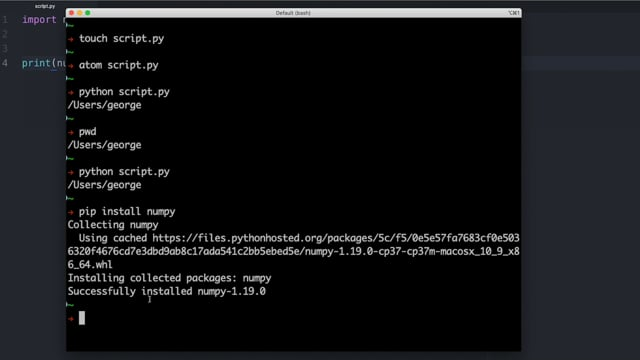Last updated on 3/1/22

## Import Python Packages and ModulesAs you have already seen, to make use of a Python module in the Python Standard Library you can use the syntax that you are already familiar with:

``````import <module>
``````

For example, if you want to use the  `os`  module from the Python Standard Library to print the current working directory of a process, you could create a file called  `script.py`  with the following contents:

``````import os

print(os.getcwd())
``````

You could then run  `script.py`  from the terminal using  `python script.py`  :

```~
→ python script.py
/Users/george
~
→```

In the script we import the entire  `os`  module and make use of a piece of its functionality by calling  `os.getcwd()` .

As an alternative to this, we can instead choose to  `import`  selected functionality from the  `os`  package as follows:

``````from os import getcwd

print(getcwd())
``````

We import third-party Python packages into Python files in the same way as we import modules from the Python Standard Library into Python files. For example, we could make use of the  `numpy`  package to round numbers up and down.

First, let’s install  `numpy`  by running  `pip install numpy`  from the command line terminal:

```→ pip install numpy
Collecting numpy
Installing collected packages: numpy
Successfully installed numpy-1.19.0
~
→```

Now, we can  `import`   `numpy`  in a Python script and use it to round some numbers up and down. Create a Python script called  `number_rounding.py`  and put the following code into your script:

``````import numpy

print(numpy.ceil(1.2))
print(numpy.ceil(1.933))
print(numpy.floor(1.2))
``````

As you can see, we  `import`  the third-party  `numpy`  Python package in the same way as we would  `import`  modules in the Python Standard Library such as  `json`  ,  `os`  ,  `datetime`  and  `re` . Running  `number_rounding.py`  should generate the following output:

```→ python number_rounding.py
2.0
2.0
1.0
~
→```

An alternative to using the  `import <package>`  syntax is to instead  `import`  everything from a package using  `from <package> import *`  . This can be useful when you are using the Python shell, because it means you can type less.

For example, let’s start by opening the Python shell by typing  `python`  in the command line terminal:

```→ python
Python 3.7.1 (default, Dec142018, 13:28:58)
[Clang 4.0.1 (tags/RELEASE_401/final)] :: Anaconda, Inc. on darwin
>>>```

Now, let’s  `import`  everything from the  `numpy`  package using  `from numpy import *`  :

```→ python
Python 3.7.1 (default,Dec142018,13:28:58)
[Clang 4.0.1 (tags/RELEASE_401/final)] :: Anaconda, Inc. on darwin
>>> from numpy import *
>>>```

Now, we can use the  `ceil()`  and  `floor()`  methods without having to prefix them with  `numpy`  :

```→ python
Python 3.7.1 (default, Dec142018, 13:28:58)
[Clang 4.0.1 (tags/RELEASE_401/final)] :: Anaconda, Inc. on darwin
>>> from numpy import *
>>> ceil(1.2)
2.0
>>> floor(1.2)
1.0```

While using  `from <package> import *`  in the Python shell is fine, it generally isn’t considered good practice in application source code. If you use  `from <package_a> import *`  and  `from <package_b> import *`  and there is a name clash between functionality in  `package_a`  and functionality in  `package_b` , then this might result in unintended consequences.

When importing packages you also have the option to  `import`  them with an alias using the command  `import <package> as <alias>` . Strangely, this is industry standard for some packages, but would be considered unconventional for other packages.

For example, it is in fact industry standard to  `import` `numpy`  using  `import numpy as np`  . If we apply this style of  `import`  to  `number_rounding.py`  , it would become:

``````import numpy as np

print(np.ceil(1.2))
print(np.ceil(1.933))
print(np.floor(1.2))
``````

It’s functionality remains unchanged, so when we run it using Python the output is exactly the same:

```→ python number_rounding.py
2.0
2.0
1.0
~
→```

Using the  `np`  alias in this case means that for large applications with lots of source code, the code becomes slightly easier to read and understand, and thus more maintainable/extendable.

You can choose to use any alias you wish, but it is generally best to use something which could be considered shorthand for the package name. Using `import numpy as apples`  wouldn’t make much sense, for example!

For a bit more information around importing Python packages, check out this screencast:Now that you have completed this chapter you should be confident with the following tasks:

• You can  `import`  Python packages into Python files in the same way as importing modules from the Python Standard Library.

• It is good practice to become familiar with, and accustomed to, a variety of methods for importing modules and packages and then choosing the most appropriate  `import`  method for your particular use case.

Now that you are more confident with importing packages and modules into Python files, let’s take a look at why versioning of packages is so important in software development.